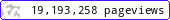•••••MadAsMaths.com :: I.Y.G.B. Practice Papers :: A Level Practice Papers 2019 Spec :: FP1 Practice Papers

I.Y.G.B. FP1 PRACTICE PAPERS

These I.Y.G.B. practice papers mostly follow the Pearson/Edexcel Syllabus introduced for teaching from 2017.

Some modules will be first examined in 2018 and some in 2019.

• Papers A to R have standard difficulty with later papers usually more difficult.
• Papers S and T are extremely hard.
• Papers U to Z are hard.

All papers have model solutions.

 fp1_a.pdffp1_a_solutions.pdffp1_b.pdffp1_b_solutions.pdffp1_c.pdffp1_c_solutions.pdffp1_d.pdffp1_d_solutions.pdffp1_e.pdffp1_e_solutions.pdffp1_f.pdffp1_f_solutions.pdffp1_g.pdffp1_g_solutions.pdffp1_h.pdffp1_h_solutions.pdffp1_i.pdffp1_i_solutions.pdffp1_j.pdffp1_j_solutions.pdffp1_k.pdffp1_k_solutions.pdffp1_l.pdffp1_l_solutions.pdffp1_m.pdffp1_m_solutions.pdffp1_n.pdffp1_n_solutions.pdffp1_o.pdffp1_o_solutions.pdffp1_p.pdffp1_p_solutions.pdffp1_q.pdffp1_q_solutions.pdffp1_r.pdffp1_r_solutions.pdffp1_s.pdffp1_s_solutions.pdffp1_t.pdffp1_t_solutions.pdffp1_u.pdffp1_u_solutions.pdffp1_v.pdffp1_v_solutions.pdffp1_w.pdffp1_w_solutions.pdffp1_x.pdffp1_x_solutions.pdffp1_y.pdffp1_y_solutions.pdffp1_z.pdffp1_z_solutions.pdf